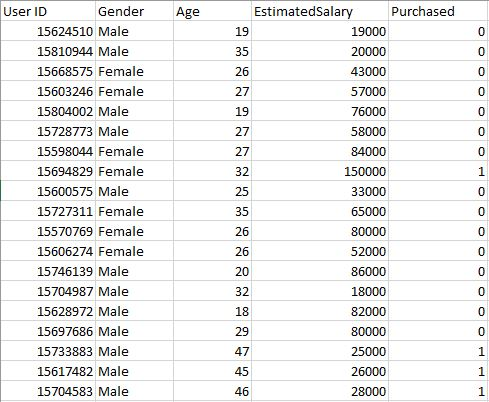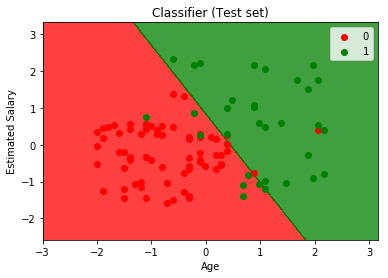# ML | Logistic Regression using Python

Prerequisite: Understanding Logistic Regression

User Database – This dataset contains information of users from a companies database. It contains information about UserID, Gender, Age, EstimatedSalary, Purchased. We are using this dataset for predicting that a user will purchase the company’s newly launched product or not.

Data – User_DataLet’s make the Logistic Regression model, predicting whether a user will purchase the product or not.

Inputing Libraries

 `import` `pandas as pd ` `import` `numpy as np ` `import` `matplotlib.pyplot as plt `

 `dataset ``=` `pd.read_csv(``'...\\User_Data.csv'``) `

Now, to predict whether a user will purchase the product or not, one needs to find out the relationship between Age and Estimated Salary. Here User ID and Gender are not important factors for finding out this.

 `# input ` `x ``=` `dataset.iloc[:, [``2``, ``3``]].values ` ` `  `# output ` `y ``=` `dataset.iloc[:, ``4``].values `

Splitting the dataset to train and test. 75% of data is used for training the model and 25% of it is used to test the performance of our model.

 `from` `sklearn.cross_validation ``import` `train_test_split ` `xtrain, xtest, ytrain, ytest ``=` `train_test_split( ` `        ``x, y, test_size ``=` `0.25``, random_state ``=` `0``) `

Now, it is very important to perform feature scaling here because Age and Estimated Salary values lie in different ranges. If we don’t scale the features then Estimated Salary feature will dominate Age feature when the model finds the nearest neighbor to a data point in data space.

 `from` `sklearn.preprocessing ``import` `StandardScaler ` `sc_x ``=` `StandardScaler() ` `xtrain ``=` `sc_x.fit_transform(xtrain)  ` `xtest ``=` `sc_x.transform(xtest) ` ` `  `print` `(xtrain[``0``:``10``, :]) `

Output :

```[[ 0.58164944 -0.88670699]
[-0.60673761  1.46173768]
[-0.01254409 -0.5677824 ]
[-0.60673761  1.89663484]
[ 1.37390747 -1.40858358]
[ 1.47293972  0.99784738]
[ 0.08648817 -0.79972756]
[-0.01254409 -0.24885782]
[-0.21060859 -0.5677824 ]
[-0.21060859 -0.19087153]]```

Here once see that Age and Estimated salary features values are sacled and now there in the -1 to 1. Hence, each feature will contribute equally in decision making i.e. finalizing the hypothesis.

Finally, we are training our Logistic Regression model.

 `from` `sklearn.linear_model ``import` `LogisticRegression ` `classifier ``=` `LogisticRegression(random_state ``=` `0``) ` `classifier.fit(xtrain, ytrain) `

After training the model, it time to use it to do prediction on testing data.

 `y_pred ``=` `classifier.predict(xtest) `

Let’s test the performance of our model – Confusion Matrix

 `from` `sklearn.metrics ``import` `confusion_matrix ` `cm ``=` `confusion_matrix(ytest, y_pred) ` ` `  `print` `(``"Confusion Matrix : \n"``, cm) `

Output :

```Confusion Matrix :
[[65  3]
[ 8 24]]```

Out of 100 :
TruePostive + TrueNegative = 65 + 24
FalsePositive + FalseNegative = 3 + 8

Performance measure – Accuracy

 `from` `sklearn.metrics ``import` `accuracy_score ` `print` `(``"Accuracy : "``, accuracy_score(ytest, y_pred)) `

Output :

`Accuracy :  0.89`

Visualizing the performance of our model.

 `from` `matplotlib.colors ``import` `ListedColormap ` `X_set, y_set ``=` `xtest, ytest ` `X1, X2 ``=` `np.meshgrid(np.arange(start ``=` `X_set[:, ``0``].``min``() ``-` `1``,  ` `                               ``stop ``=` `X_set[:, ``0``].``max``() ``+` `1``, step ``=` `0.01``), ` `                     ``np.arange(start ``=` `X_set[:, ``1``].``min``() ``-` `1``,  ` `                               ``stop ``=` `X_set[:, ``1``].``max``() ``+` `1``, step ``=` `0.01``)) ` ` `  `plt.contourf(X1, X2, classifier.predict( ` `             ``np.array([X1.ravel(), X2.ravel()]).T).reshape( ` `             ``X1.shape), alpha ``=` `0.75``, cmap ``=` `ListedColormap((``'red'``, ``'green'``))) ` ` `  `plt.xlim(X1.``min``(), X1.``max``()) ` `plt.ylim(X2.``min``(), X2.``max``()) ` ` `  `for` `i, j ``in` `enumerate``(np.unique(y_set)): ` `    ``plt.scatter(X_set[y_set ``=``=` `j, ``0``], X_set[y_set ``=``=` `j, ``1``], ` `                ``c ``=` `ListedColormap((``'red'``, ``'green'``))(i), label ``=` `j) ` `     `  `plt.title(``'Classifier (Test set)'``) ` `plt.xlabel(``'Age'``) ` `plt.ylabel(``'Estimated Salary'``) ` `plt.legend() ` `plt.show() `

Output :Analysing the performance measures – accuracy and confusion matrix and the graph, we can clearly say that our model is performing really good.

My Personal Notes arrow_drop_upIf you like GeeksforGeeks and would like to contribute, you can also write an article using contribute.geeksforgeeks.org or mail your article to contribute@geeksforgeeks.org. See your article appearing on the GeeksforGeeks main page and help other Geeks.

Please Improve this article if you find anything incorrect by clicking on the "Improve Article" button below.

Article Tags :
Practice Tags :

3

Please write to us at contribute@geeksforgeeks.org to report any issue with the above content.